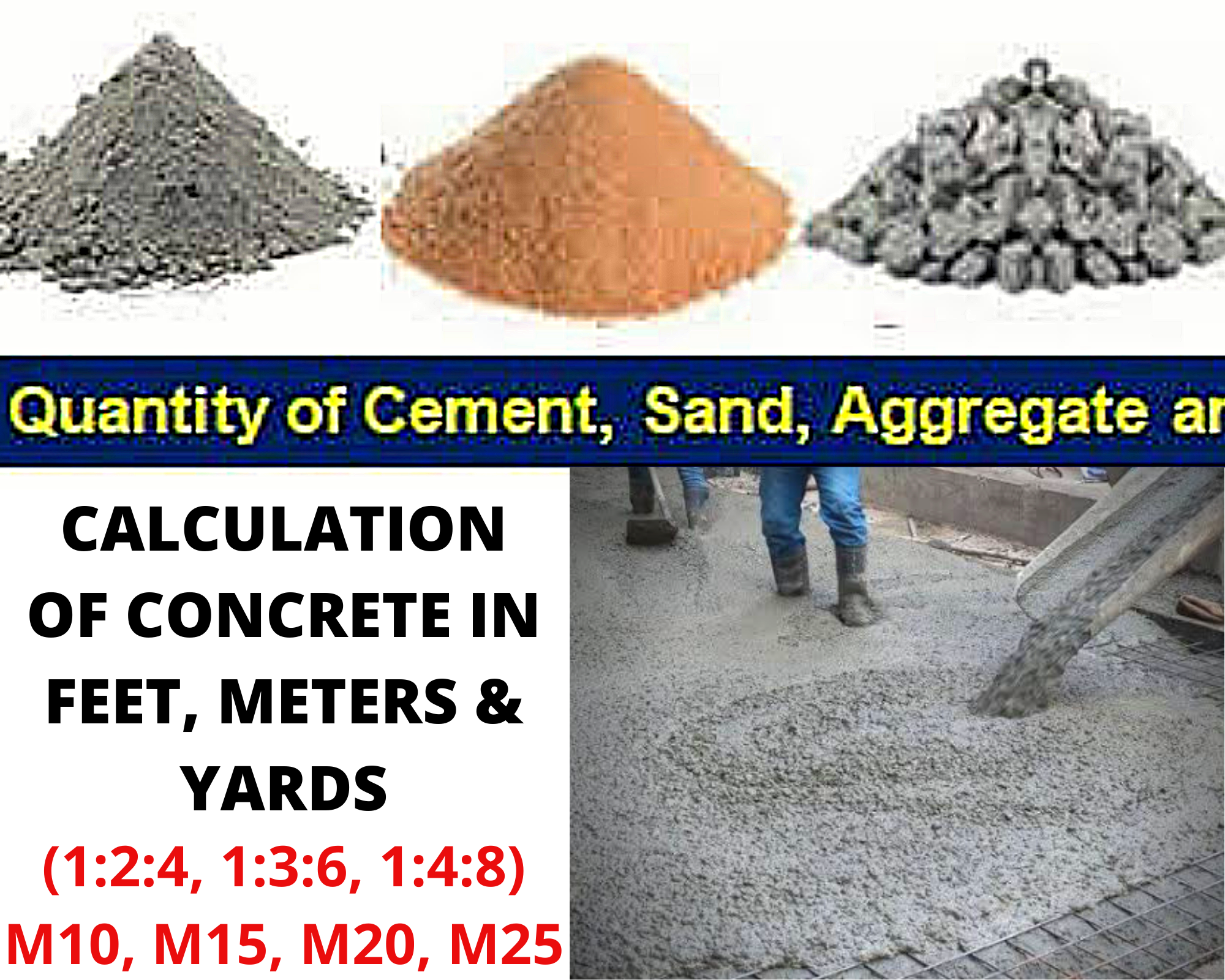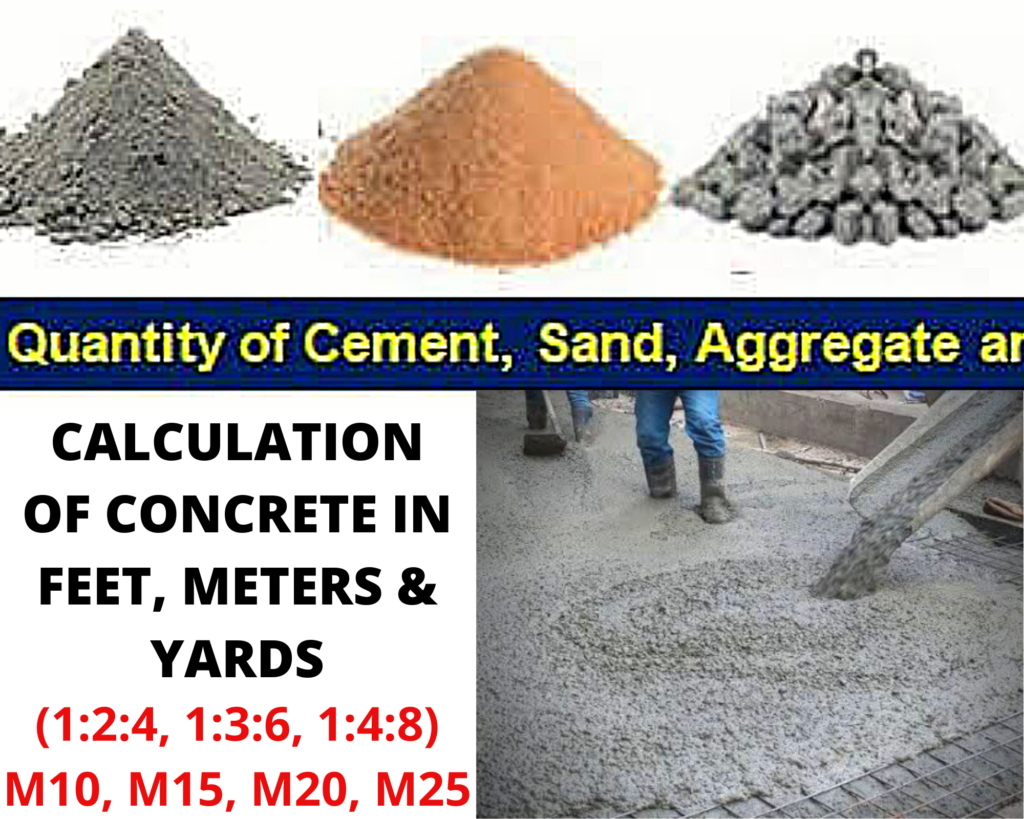# How to calculate cement, sand, aggregate in concrete?

There’re many types of concrete and grades of concrete (e.g. Ready mix, Precast, Reinforced conc). In Calculation of concrete, first of all Multiply Length and Width & Hight to get Volume. It is same as Plaster/mortar calculation.

Let suppose a slab of single story house which has 50 feet length and 25 feet width.

Length= 50 ft

Width= 25 ft

Area of slab = L×W

Area of slab = 50’×25′ = 1250 sft or 138.9 sqyd

Thickness of slab = 6 inch = 0.5 ft (That commonly used)

Wet Volume of Concrete = Area × Thickness of slab

Wet Volume=1250×(6/12) = 625 Cft

• 6 divided by 12 to convert inch into foot

Dry Volume of concrete = 625×1.54= 962.5 Cft or 27.25 cu.m (35.31cft = 1 cu.m)

“It is compulsory to multiply with 1.54, to convert wet volume of concrete into dry volume (because addition & evaporation of water cause shrinkage in concrete mix, which ultimately decrease in volume).”

Dry volume means you need 962.5 Cft concrete for 25’x50′ slab.

## How to calculate Yards of concrete?

1. Calculate volume in Feet
2. Multiply with 1.54 (to convert into dry volume)
3. Divide by 27 to convert into Cubic Yards.calculate cement, sand, aggregate in concrete

Now we will use dry volume in concrete calculation to get Quantity of Cement, Sand & Aggregate for 1:2:4 concrete mix calculation.

Ratio of concrete mix (Grade M15) = 1:2:4

Sum of ratio = 7

## Cement quantity in Concrete:

1. Quantity of Cement = (1÷7)×962.5 = 137.5 Cft
2. Cement = (1÷7)×27.25 = 3.9 Cum

Density of cement = 1440kg/m3 or 40.77kg/cft

• Multiply cft with 40.77 & cum with 1440 to get quantity of cement in KGs.

Cft>>> 137.5×40.77= 5606 Kg

Cum>>> 3.9×1440= 5606 kg

How to calculate cement bags in concrete? To find cement Bags divide by 50 (50 kg in one bag of cement).

5606/50 = 112 bags of cement

Or directly calculate cement bags from Volume:

Volume of cement bag= 0.034cum or 1.226cft

Cft>>> 137.5÷1.226 = 112 Bags

Read also Plaster/mortar calculation.

## Calculation of sand in Concrete:

1. Quantity of Sand = (2÷7)×962.5 = 275 Cft
2. Sand = (2÷7)×27.25 = 7.78 Cu.m

Density of sand is 1600kg/m3 OR 45.30 kg/cft

Read also Brick calculation.

## Calculateaggregate quantity in Concrete:

1. Quantity of Aggregate = (4÷7)×962.5 = 550Cft
2. Aggregate = (4÷7)×27.25 = 15.57 Cu.m

## Calculation of Water Cement Ratio in concrete:

1. Water cement ratio means quantity of water against cement (in kgs).
2. W-C Ratio = 50% × Quantity of cement (kg)
3. Water= 5606×0.50 = 2803 liters.

50% of water quantity is commonly used in ordinary concrete mix , but it can be b/w 22% to 50%.

Share